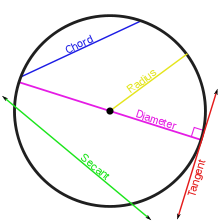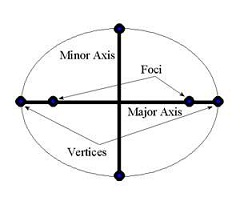#Difference Between Similar Terms and Objects

# Difference Between Circle and Ellipse

Circle vs Ellipse

A circle and an ellipse are sections of a cone. The cone has four sections; circle, ellipse, hyperbola, and parabola. The conic section is a section which is obtained when a cone is cut by a plane. The cone has a base, an axis, and two sides. Circles and ellipses are differentiated on the basis of the angle of intersection between the plane and the axis of the cone. Both circles and ellipses are closed curves.
Circle
A circle is basically a line which forms a closed loop. In a circle, the set of points are equidistant from the center. It is a closed curve which has an interior and an exterior. It is attained when the plane intersects the right circular cone perpendicular to the cone axis. A circle is a two-dimensional figure whereas a disk, which is also attained in the same way as a circle, is a three-dimensional figure meaning the interior of the circle is also included in the disk. The eccentricity of a circle is zero.

Center: The point inside the circle from which all points on the circle are equidistant.
Diameter: It is the distance across the circle through the center.
Radius: The radius is the distance between the center to any point on the circle; it is half of the diameter.
Circumference: The distance around the circle is called the circumference.
Chord: When a line segment links any two points on a circle, it is called a chord. When this chord passes through the center, it becomes the diameter.
Tangent: A tangent is a straight line passing a circle and touching it at just one point.
Secant: A secant is a straight line which cuts the circle at two points.
Arc: Any part of the circumference of a circle is called an arc.
Sector: A region inside the circle bound by one arc and two radii is called a sector.
Segment: A region bound by an arc and a chord is called a segment.
Pi: The value of pi is approximately 3.142. When the circumference of a circle is divided by its diameter, we get the same number always. This number is called pi.http://en.wikipedia.org/wiki/Circle

Ellipse
An ellipse is attained when the plane cuts through the cone orthogonally through the axis of the cone. A circle is a special ellipse. In an ellipse, the distance of the locus of all points on the plane to two fixed points (foci) always adds to the same constant.
Major and minor axis: It is the diameters of an ellipse. The major axis is the longer diameter and the minor axis is the shorter diameter.
Semi-major and semi-minor axis: It is the distance between the center and the longest point and the center and the shortest point on the ellipse.
Foci: Two fixed points in the interior of the ellipse are called foci.
Other elements of an ellipse are the same as a circle like chord, segment, sector, etc.
The eccentricity of an ellipse is always between 0 and 1.Source: http://freemathdictionary.com/ee/ellipse/
Summary:
A circle is a special case of an ellipse where the two foci or fixed points inside the ellipse are coincident and the eccentricity is zero.
In a circle, all the diameters are the same size, but in an ellipse there are major and minor axes which are of different lengths.
Similarly, the radii of a circle are all the same length. In an ellipse, the semi-major axis and semi-minor axis are of different lengths.
The eccentricity of a circle is always 1; the eccentricity of an ellipse is 0 to 1.

Latest posts by Nimisha Kaushik (see all)

### Search DifferenceBetween.net :

Custom Search

Help us improve. Rate this post!Loading...Email This Post : If you like this article or our site. Please spread the word. Share it with your friends/family.

Please note: comment moderation is enabled and may delay your comment. There is no need to resubmit your comment.

Articles on DifferenceBetween.net are general information, and are not intended to substitute for professional advice. The information is "AS IS", "WITH ALL FAULTS". User assumes all risk of use, damage, or injury. You agree that we have no liability for any damages.